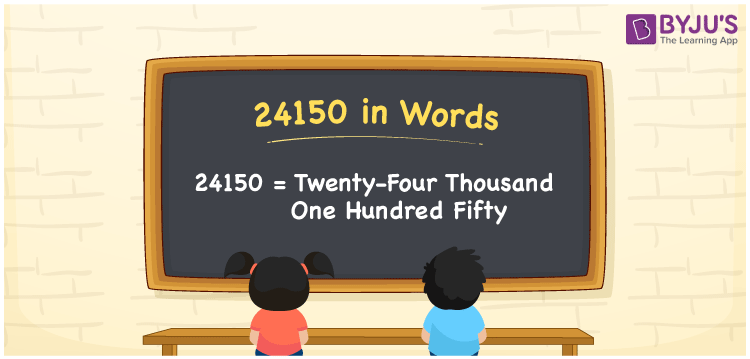# 24150 in Words

We can write 24150 in words is written as Twenty-four thousand one hundred fifty. If you purchased a new home theatre projector that costs Rs. 24150, then you can say, “I bought a new home theatre projector worth Twenty-four thousand one hundred fifty rupees”. In this article, you will learn how to write the number 24150 in words with the help of a place value chart.

 24150 in words Twenty-four thousand one hundred fifty Twenty-four thousand one hundred fifty in Numbers 24150

## 24150 in English Words

Generally, we use the English alphabet to express numbers in words. Thus, we spell 24150 in English words as “Twenty-four thousand one hundred fifty”.## How to Write 24150 in Words?

The number 24150 has five digits so let’s make a place value chart with five columns to convert the number 24150 to word form.

 Ten thousands Thousands Hundreds Tens Ones 2 4 1 5 0

Here, ones = 0, tens = 5, hundreds = 1, thousands = 4, ten thousands = 2

By expanding these numbers, we get;

2 × Ten thousand + 4 × Thousand + 1 × Hundred + 5 × Ten + 0 × One

= 2 × 10000 + 4 × 1000 + 1 × 100 + 5 × 10 + 0 × 1

= 20000 + 4000 + 100 + 50

= Twenty Thousand + Four thousand + One hundred + Fifty

= Twenty-four thousand + One hundred fifty

= Twenty-four thousand one hundred fifty

Hence, 24150 in words = Twenty-four thousand one hundred fifty.

As we know, 24150 is a natural number that is the successor of 24149 and the predecessor of 24151.

24150 in words – Twenty-four thousand one hundred fifty

Is 24150 an odd number? – No

Is 24150 an even number? – Yes

Is 24150 a prime number? – No

Is 24150 a composite number? – Yes

Is 24150 a perfect square number? – No

Is 24150 a perfect cube number? – No

## Frequently Asked Questions on 24150 in Words

Q1

### How do you write 24150 in words?

The number 24150 can be written in words as Twenty-four thousand one hundred fifty.
Q2

### How do you write Rs. 24150 in words on a cheque?

On a cheque, we generally write Rs. 24150 in words as “Twenty-four thousand one hundred fifty rupees only”.
Q3

### Express the number 24150 in word form.

We express the number 24150 in word form as Twenty-four thousand one hundred fifty.